# Area

The area of an 2D shape would be the total size of its surface. Area is measured in square units such as square centimeters ( $$cm^2$$ ), square inches ( $$in^2$$ ) etc..

## Area of Common Shapes

Let's look at how to calculate the area of the shapes below:

### Triangle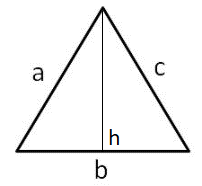The area of a triangle with height h and base b, is calculated by:

$$A = \cfrac{1}{2} b h$$

### Square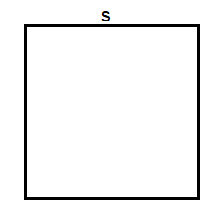The area of a square with length s is calculated by:

$$A = s^2$$

### Rectangle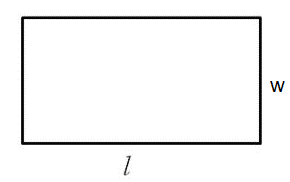The area of a rectangle with length l and base b, is calculated by:

$$A = b \cdot l$$

### Circle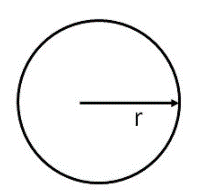The area of a circle with radius r is calculated by:

$$A = \pi r^2$$

### Trapezoid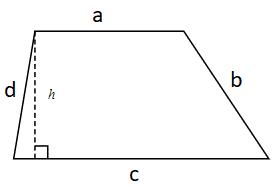The area of a trapezoid with sides a, b, c, d and height h is calculated by:

$$A = \cfrac{1}{2} (a + c ) h$$

### Parallelogram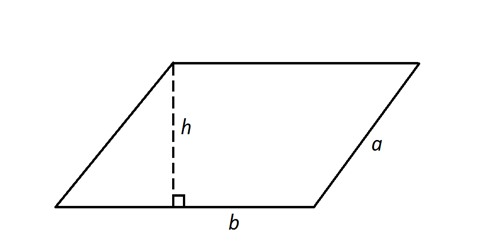The area of a parallelogram with side length a, base length b and height h is calculated by:

$$A = b \cdot h$$

The area of a rectangle is 28 square meters. The length is 7 meters. What is the width of the rectangle?

The diameter of a round party size pizza at Gino's Pizza is 20 inches. What is the area of the delicious pizza? If the pizza is cut into 16 slices, what is the area of a single slice?